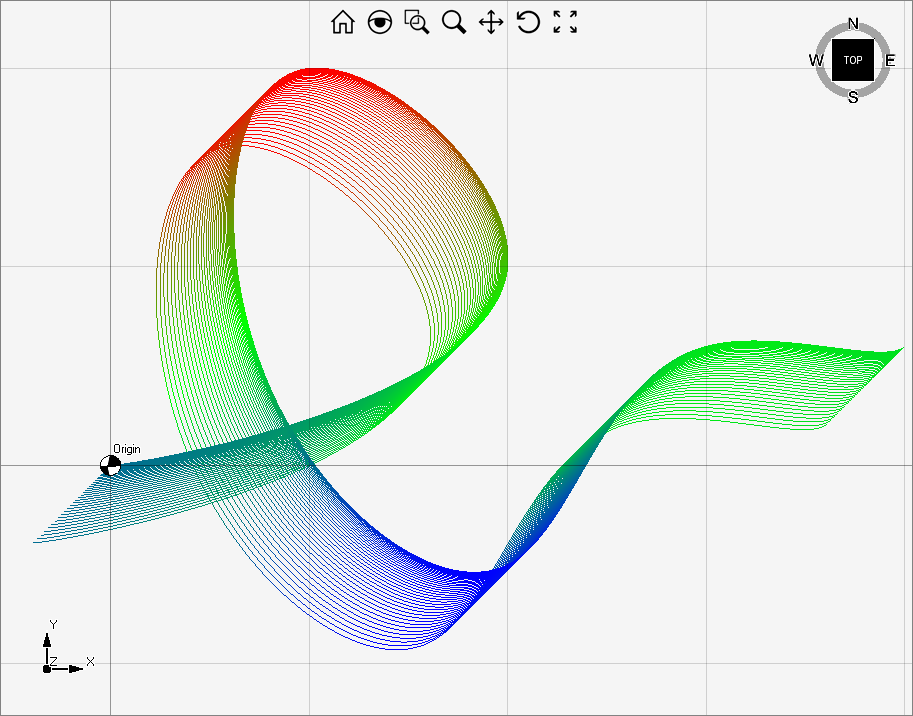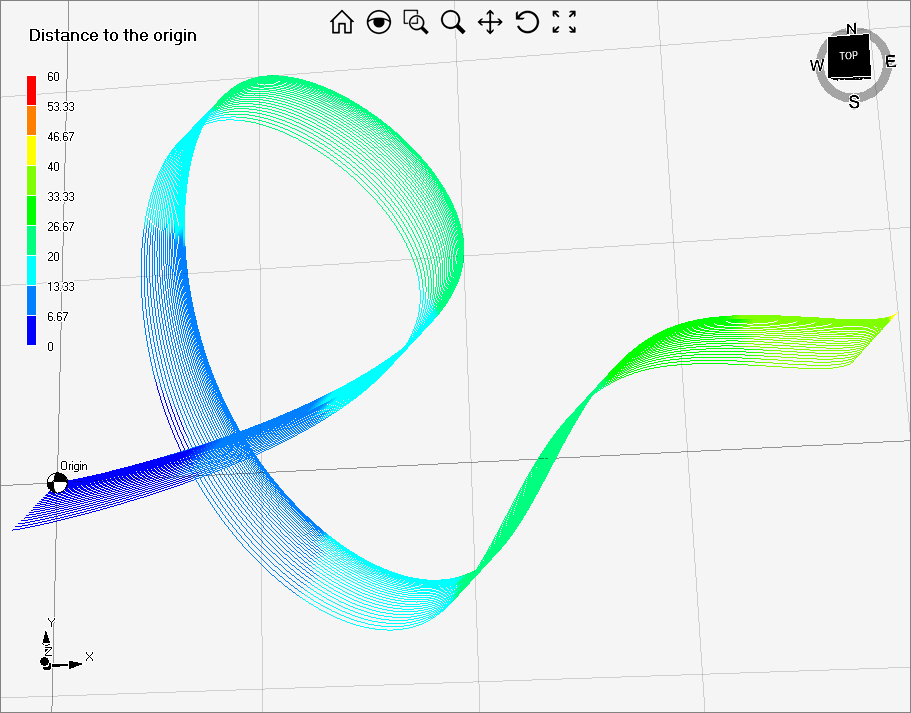# Create streamline-like curve

With the first approach,  you will have full control over the streamlines colors since you can set the RBG color of each the PointCloud's vertexes.

With the second approach, you can choose colors by means of a Color Table. This is a tool that allows you to easily create a map between numerical values and colors.The following code shows how to set the color based on the y-coordinates on the streamline's points, but you can customize it following your own rule.

``````
PointCloud pc;
List<Point3D> interPoints = new List<Point3D> { Point3D.Origin, new Point3D(20, 10, 0), new Point3D(10, 20, 0), new Point3D(8, 4, 0), new Point3D(20, -5, 0), new Point3D(30, 6, 0), new Point3D(40, 6, 0) };
Curve curve = Curve.CubicSplineInterpolation(interPoints, new Vector3D(1, 0, 0), new Vector3D(1, 1, 0));
curve.Regen(0.001);
double maximum;
double minimum;
double ratio;
int R, G, B;
for (int j = 0; j < 40; j++)
{
curve.Translate(-j * 0.005, -j * 0.005, 0);

curve.Regen(0.001);
pc = new PointCloud(curve.Vertices.Length, 1, PointCloud.natureType.Multicolor);
//set the color
for (int i = 0; i < curve.Vertices.Length; i++)
{
minimum = curve.BoxMin.Y;
maximum = curve.BoxMax.Y;
ratio = 2 * (curve.Vertices[i].Y - minimum) / (maximum - minimum);
B = (int)(Utility.Max(0, 255 * (1 - ratio), 0));
R = (int)(Utility.Max(0, 255 * (ratio - 1), 0));
G = 255 - B - R;
pc.Vertices[i] = new PointRGB(curve.Vertices[i].X, curve.Vertices[i].Y, curve.Vertices[i].Z, Color.FromArgb(0, R, G, B));

}
pc.DrawingStyle = PointCloud.drawingStyleType.LineStrip;
}

``````

The following code creates streamlines with colors that depend on the distance between the streamline's point and the origin.

The minimum and maximum values of the Legend should form an interval that contains the range of the numerical function used to set colors.``````
PointCloud pc;
List<Point3D> interPoints = new List<Point3D> { Point3D.Origin, new Point3D(20, 10, 0), new Point3D(10, 20, 0), new Point3D(8, 4, 0), new Point3D(20, -5, 0), new Point3D(30, 6, 0), new Point3D(40, 6, 0) };
Curve curve = Curve.CubicSplineInterpolation(interPoints, new Vector3D(1, 0, 0), new Vector3D(1, 1, 0));
Legend legend = model1.Legends;
model1.Legends.Title = "Distance to the origin";
model1.Legends.Subtitle = "";
model1.Legends.Visible = true;
legend.ColorTable = Legend.RedToBlue9;
legend.Min = 0;
legend.Max=60;
for (int j = 0; j < 30; j++)
{

pc = new PointCloud(curve.Vertices.Length, 1, PointCloud.natureType.Multicolor);
curve.Translate(-j * 0.005, -j * 0.005, 0);
curve.Regen(0.01);
//set the color
for (int i = 0; i < curve.Vertices.Length; i++)
{
//set the color based on the distance to the origin
int colorID = (int)((curve.Vertices[i].DistanceTo(Point3D.Origin) - legend.Min) * (legend.ColorTable.Length) / (legend.Max - legend.Min));
pc.Vertices[i] = new PointRGB(
curve.Vertices[i].X,
curve.Vertices[i].Y,
curve.Vertices[i].Z,
legend.ColorTable[colorID].R,
legend.ColorTable[colorID].G,
legend.ColorTable[colorID].B);
}
pc.DrawingStyle = PointCloud.drawingStyleType.LineStrip;